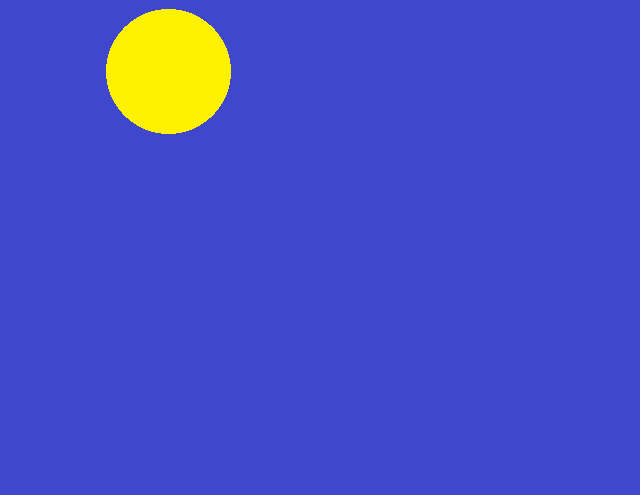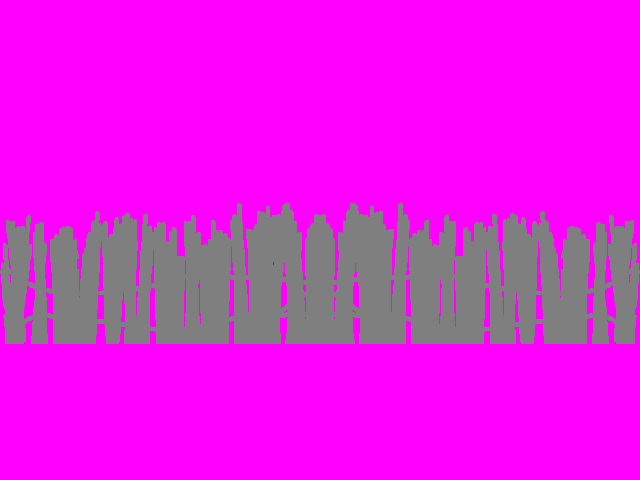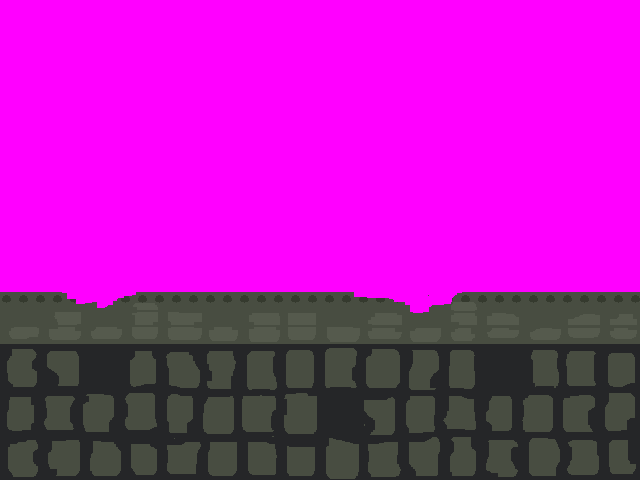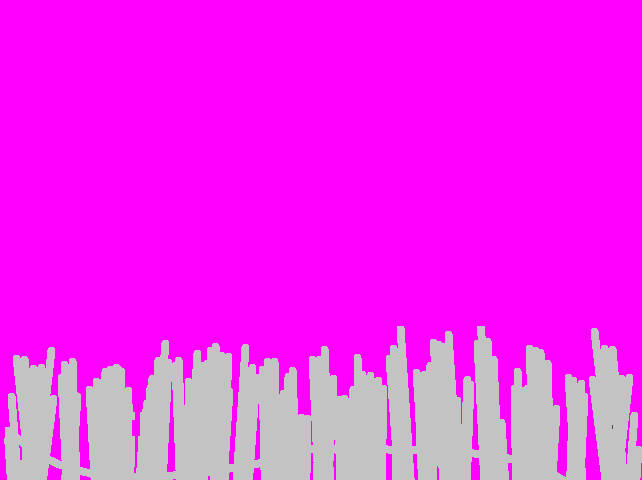## Retro parallax scrolling

Game development specific discussions.
BasicCoder2
Posts: 3620
Joined: Jan 01, 2009 7:03
Location: Australia

### Retro parallax scrolling

viewtopic.php?f=7&t=28703
Inspired by the above example I thought maybe a retro example as well.
I drew similar graphics as the above but with MSPAINT.

You can scroll left or right using the arrow keys.

The example below also uses FBImage in order to load the .png files.
FBImage.bi

Code: Select all

`#ifndef __FBImage_bi__#define __FBImage_bi__#ifdef __FB_WIN32__# libpath "lib/win"#else# libpath "lib/win"#endif#ifndef __FB_64BIT__# inclib "FBImage-32-static"#else# inclib "FBImage-64-static"#endif' Load BMP, PNG, JPG, TGA, DDS from file or memory as FBImage' screenres 640,480,32 ' <--- RGBA' var jpg = LoadRGBAFile("test_rgb.jpg")' put (0,0),jpg,PSET'' var png = LoadRGBAFile("test_rgba.png")' put (256,0),png,ALPHA' var img = LoadRGBAFile("filenotfound.xxx")' if img=0 then'   print "error: loading filenotfound.xxx " & *GetLastResult()' end if' Save RGB image as PNG' var ok = SavePNGFile(img,"test_rgb.png")' Save RGBA image as PNG' var ok = SavePNGFile(img,"test_rgba.png",true)extern "C"declare function LoadRGBAFile(byval filename as const zstring ptr) as any ptrdeclare function LoadRGBAMemory(byval buffer as const any ptr, byval buffersize as long) as any ptrdeclare function GetLastResult() as const zstring ptrdeclare function SavePNGFile (byval img as any ptr, byval filename as const zstring ptr,byval saveAlpha as boolean=false) as booleanend extern' load (32bit) RGBA image and convert it for 16 bit RGB modefunction Load16BitRGB(filename as const zstring ptr) as any ptr  #define RGB16(_r,_g,_b) ((((_b) shr 3) shl 11) or (((_g) shr 2) shl 5) or ((_r) shr 3))  var imgSrc = LoadRGBAFile(filename)  if imgSrc=0 then return 0  dim as integer w,h,spitch,dpitch  dim as ubyte ptr s  imageinfo imgSrc,w,h,,spitch,s  var imgDst = ImageCreate(w,h,0,16)  dim as ushort ptr d  imageinfo imgDst,,,,dpitch,d   dpitch shr= 1 ' pitch in bytes to pitch in pixels  for y as integer =1 to h    dim as integer i    for x as integer =0 to w-1      d[x] = RGB16(s[i],s[i+1],s[i+2])       i+=4 ' next source pixel    next    s+=spitch ' next src row    d+=dpitch ' next dst row  next  ImageDestroy imgSrc  return imgDst  #undef RGB16end functionnamespace Base64  static as string*64 B64 = "ABCDEFGHIJKLMNOPQRSTUVWXYZ" _                          & "abcdefghijklmnopqrstuvwxyz" _                          & "0123456789+/"  Function EncodeMemory(buffer as any ptr,size as long) As String    #define E0 (S[j] shr 2)    #define E1 (((S[j] and &H03) shl 4) + (S[j+1] shr 4))    #define E2 (((S[j+1] and &H0F) shl 2) + (S[j+2] shr 6))    #define E3 (S[j+2] and &H3F)    dim as long nChars = size    if nChars=0 then return ""    dim as ubyte ptr S=buffer    dim as long j,k,m = nChars mod 3    dim as string r=string(((nChars+2)\3)*4,"=")    nChars-= (m+1)    For j = 0 To nChars Step 3      r[k]=B64[e0] : r[k+1]=B64[e1] : r[k+2]=B64[e2] : r[k+3]=B64[e3]:k+=4    Next    if m then      r[k]=B64[e0] : r[k+1]=B64[e1] : r[k+3]=61      If m = 2 Then r[k+2]=B64[e2] Else  r[k+2]=61    end if    return r    #undef E0    #undef E1    #undef E2    #undef E3  End Function  Function DecodeMemory(s As String,byref nBytes as integer) As any ptr    #define P0(p) instr(B64,chr(s[n+p]))-1    dim as long nChars=Len(s)    if nChars<1 then return 0    nBytes=nChars : nChars-=1    dim as ubyte ptr O,buffer=callocate(nBytes)    O=buffer    for n As long = 0 To nChars Step 4      var b = P0(1), c = P0(2), d = P0(3)      if b>-1 then        var a = P0(0) : *O = (a shl 2 + b shr 4) : O+=1      end if      if c>-1 then *O = (b shl 4 + c shr 2) : O+=1      if d>-1 then *O = (c shl 6 + d) : O+=1    next    return buffer    #undef P0  end functionend namespace  #endif ' __FBImage_bi__`

DEMO

Code: Select all

`chdir exepath()#include once "FBImage.bi"' dodicat's regulate functionFunction Regulate(Byval MyFps As Integer,Byref fps As Integer) As Integer    Static As Double timervalue,lastsleeptime,t3,frames    var t=Timer    frames+=1    If (t-t3)>=1 Then t3=t:fps=frames:frames=0    Var sleeptime=lastsleeptime+((1/myfps)-T+timervalue)*1000    If sleeptime<1 Then sleeptime=1    lastsleeptime=sleeptime    timervalue=T    Return sleeptimeEnd FunctionConst SCRW = 640, SCRH = 480Screenres SCRW,SCRH, 32dim shared as any ptr images(0 to 4)For i as integer = 0 To 4    images(i) = imagecreate(SCRW,SCRH)    'bload "pp" & i + 1 & ".bmp",images(i)    images(i) = LoadRGBAFile("pp" & i + 1 & ".png")Nextdim shared as integer px(0 to 4)dim shared as integer xd(0 to 4)for i as integer = 0 to 4    px(i)=0    xd(i)=inext isub drawBackground()    screenlock    cls    for i as integer = 0 to 4        put (0,0),images(i),(px(i),0)-(SCRW-1,SCRH-1),trans        put (SCRW-1-px(i),0),images(i),(0,0)-(px(i)-1,SCRH-1),trans    next i    screenunlockend subDim As Integer fpsfps = 60Do    drawBackground()    if multikey(&H4D) then           for i as integer = 0 to 4            px(i) = px(i) + xd(i)  'update shift position            if px(i) > SCRW-1 then px(i) = 0        next i    end if       if multikey(&H4B) then           for i as integer = 0 to 4            px(i) = px(i) - xd(i)  'update shift position            if px(i) <0 then px(i) = SCRW-1        next i    end if       Sleep regulate(60,fps),1   Loop Until multikey(&H01)`Last edited by BasicCoder2 on Jul 23, 2020 17:38, edited 2 times in total.
UEZ
Posts: 663
Joined: May 05, 2017 19:59
Location: Germany

### Re: Retro parallax scrolling

@BasicCoder2: looks nice but why didn't you use the images I've zipped? The original images are in one big PNG image and I had cut them out and saved it as 32-bit bmp using GIMP. This 32-bit bmp format is support by FB.

Btw, the Demo code above is posted twice.

What a pitty that FB has no built-in functions for the popular image formats to load / save them.

@SARG: GAS64 has a problem to compile this.

Command Line:
C:\...\FreeBASIC64\fbc64_gas64.exe -m "C:\...\Retro parallax scrolling\Demo.bas" -v -s gui -x "C:\...\Retro parallax scrolling\Demo.exe"

Error output:

Code: Select all

`Retro parallax scrolling\Demo.a64: Assembler messages:Retro parallax scrolling\Demo.a64:926: Error: invalid use of registerRetro parallax scrolling\Demo.a64:953: Error: invalid use of registerRetro parallax scrolling\Demo.a64:988: Error: invalid use of register`
BasicCoder2
Posts: 3620
Joined: Jan 01, 2009 7:03
Location: Australia

### Re: Retro parallax scrolling

@UEZ
One reason I didn't use the images you zipped was because they came in different sizes and I could not modify and save them with MSPaint without losing transparency. Also I like to draw my own images as the stock images never fit any game idea. They are fine for a demo but not if you wanted to write your own game. I had intended adding a character to run and jump along the wall under user control.

The problem with built in functions is that they bloat up the core functions common to all languages. Personally I have no problem with just including an appropriate library of functions or objects for a particular project. The main problem I had with C++ was the convoluted messing about required to install the library so you could simply include it in your own programs.
SARG
Posts: 1186
Joined: May 27, 2005 7:15
Location: FRANCE

### Re: Retro parallax scrolling

UEZ wrote:@SARG: GAS64 has a problem to compile this.
@UEZ Thanks. One variable is named 'DX' so not good for the assembler... It's allowed in the current version but I did a mistake when compiling or copying files. See the usual thread.
Off topic.... Sorry BasicCoder2.
BasicCoder2
Posts: 3620
Joined: Jan 01, 2009 7:03
Location: Australia

### Re: Retro parallax scrolling

@SARG
Not off topic really as it was an issue with the posted code.
In fact I now remember the issue with dx and assembler and used to use xd instead but then later thought it had been fixed.
See you have now fixed it? Anyway I have just changed dx to xd in the first post.